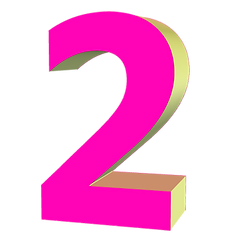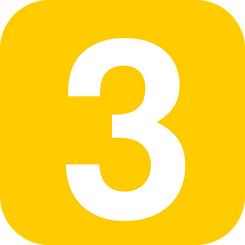# Numbers PNG images

Browse through our complete collection of number, free PNG images only on FreePNGs. We upload more free pngs weekly. Check out Snipstock for more images.

We currently have 433 number free PNGs

## ​

A number is a mathematical object used to count, measure and also label. The original examples are the natural numbers 1, 2, 3, 4 and so forth. A notational symbol that represents a number is called a numeral. In addition to their use in counting and measuring, numerals are often used for labels (as with telephone numbers), for ordering (as with serial numbers), and for codes (as with ISBNs). In common usage, number may refer to a symbol, a word, or a mathematical abstraction.

In mathematics, the notion of number has been extended over the centuries to include 0, negative numbers, rational numbers such as 1/2 and −2/3, real numbers such as √2 and π, and complex numbers, which extend the real numbers by adding a square root of −1. Calculations with numbers are done with arithmetical operations, the most familiar being addition, subtraction, multiplication, division, and exponentiation. Their study or usage is called arithmetic. The same term may also refer to number theory, the study of the properties of numbers.

Besides their practical uses, numbers have cultural significance throughout the world. For example, in Western society, the number 13 is regarded as unlucky, and "a million" may signify "a lot." Though it is now regarded as pseudoscience, numerology, the belief in a mystical significance of numbers, permeated ancient and medieval thought. Numerology heavily influenced the development of Greek mathematics, stimulating the investigation of many problems in number theory which are still of interest today.

During the 19th century, mathematicians began to develop many different abstractions which share certain properties of numbers and may be seen as extending the concept. Among the first were the hypercomplex numbers, which consist of various extensions or modifications of the complex number system. Today, number systems are considered important special examples of much more general categories such as rings and fields, and the application of the term "number" is a matter of convention, without fundamental significance.